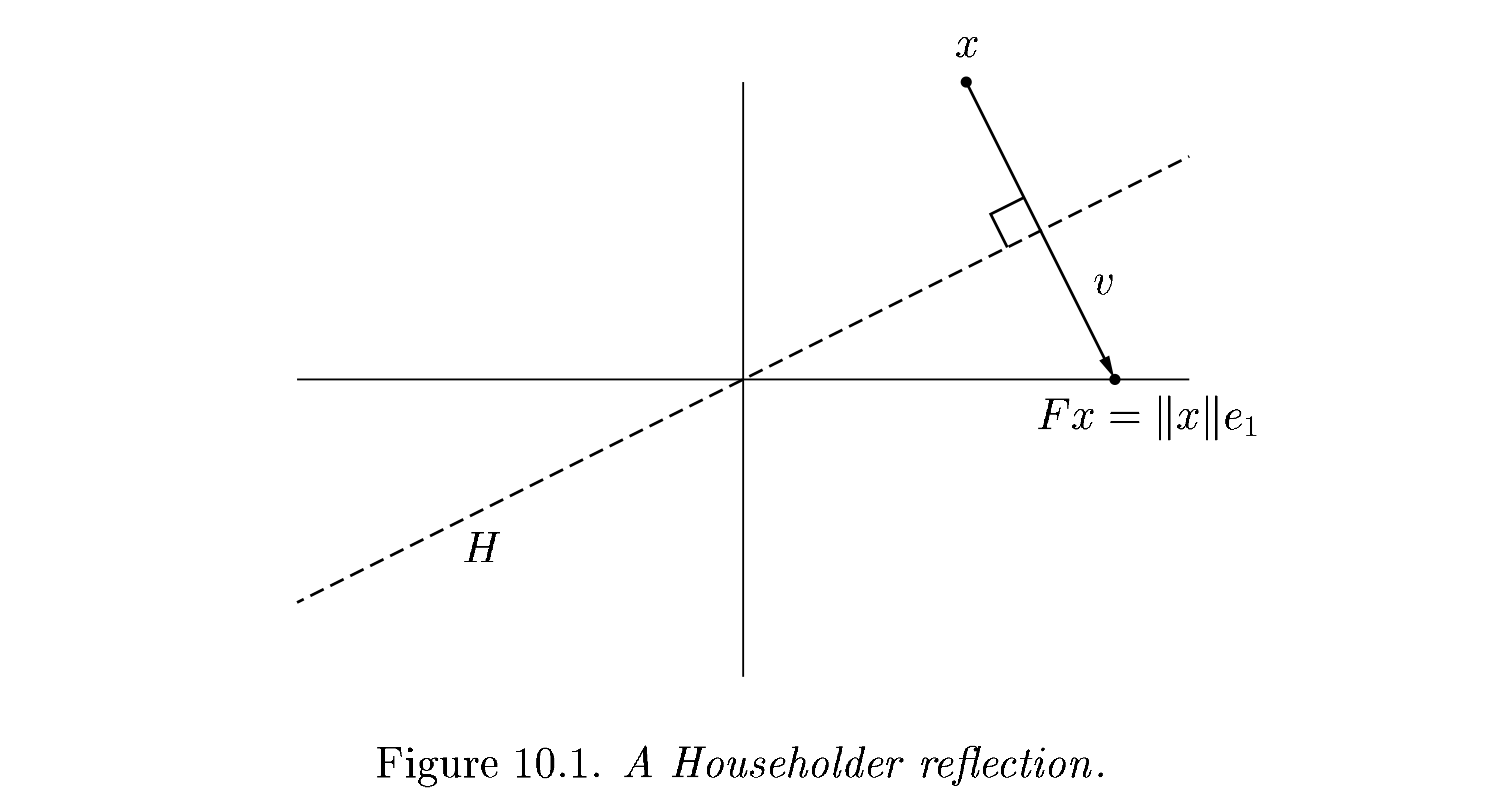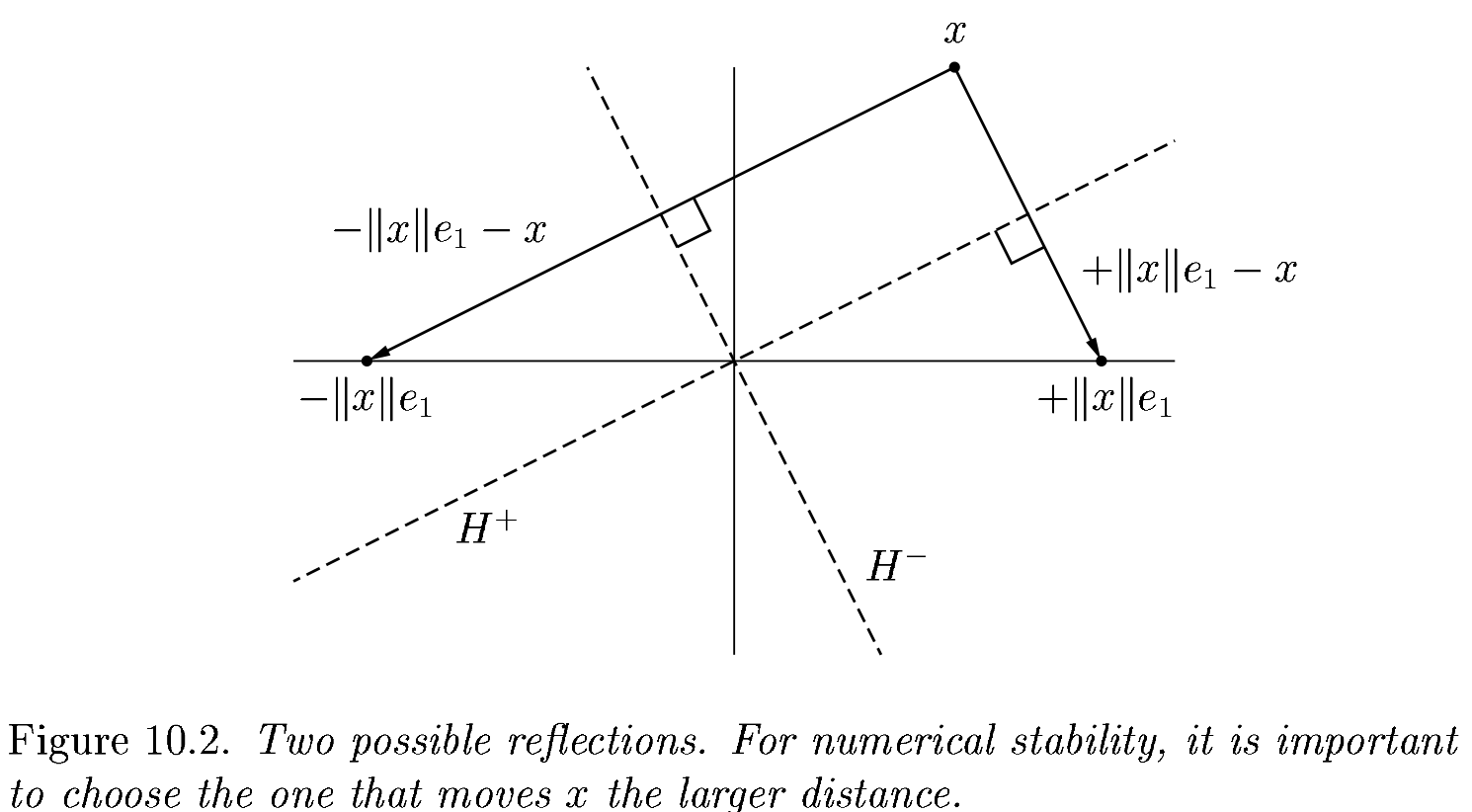# 2022-02-21 QR Retrospective¶

## Last time¶

• Performance strategies

• Right vs left-looking algorithms

• Elementary reflectors

## Today¶

• Householder QR

• Comparison of interfaces

• Profiling

• Cholesky QR

using LinearAlgebra
using Plots
default(linewidth=4, legendfontsize=12)

function vander(x, k=nothing)
if isnothing(k)
k = length(x)
end
m = length(x)
V = ones(m, k)
for j in 2:k
V[:, j] = V[:, j-1] .* x
end
V
end

function gram_schmidt_classical(A)
m, n = size(A)
Q = zeros(m, n)
R = zeros(n, n)
for j in 1:n
v = A[:,j]
R[1:j-1,j] = Q[:,1:j-1]' * v
v -= Q[:,1:j-1] * R[1:j-1,j]
R[j,j] = norm(v)
Q[:,j] = v / R[j,j]
end
Q, R
end

gram_schmidt_classical (generic function with 1 method)


# Householder QR¶

Gram-Schmidt constructed a triangular matrix $$R$$ to orthogonalize $$A$$ into $$Q$$. Each step was a projector, which is a rank-deficient operation. Householder uses orthogonal transformations (reflectors) to triangularize.

$\underbrace{Q_{n} \dotsb Q_1}_{Q^T} A = R$

The structure of the algorithm is

$\begin{split} \underbrace{\begin{bmatrix} * & * & * \\ * & * & * \\ * & * & * \\ * & * & * \\ * & * & * \\ \end{bmatrix}}_{A} \to \underbrace{\begin{bmatrix} * & * & * \\ 0 & * & * \\ 0 & * & * \\ 0 & * & * \\ 0 & * & * \\ \end{bmatrix}}_{Q_1 A} \to \underbrace{\begin{bmatrix} * & * & * \\ 0 & * & * \\ 0 & 0 & * \\ 0 & 0 & * \\ 0 & 0 & * \\ \end{bmatrix}}_{Q_2 Q_1 A} \to \underbrace{\begin{bmatrix} * & * & * \\ 0 & * & * \\ 0 & 0 & * \\ 0 & 0 & 0 \\ 0 & 0 & 0 \\ \end{bmatrix}}_{Q_3 Q_2 Q_1 A} \end{split}$

# Constructing the $$Q_j$$¶

$\underbrace{Q_{n} \dotsb Q_1}_{Q^T} A = R$

Each of our $$Q_j$$ will have the form

$\begin{split}Q_j = \begin{bmatrix} I_i & 0 \\ 0 & F \end{bmatrix}\end{split}$
where $$F$$ is a “reflection” that achieves
$\begin{split} F x = \begin{bmatrix} \lVert x \rVert \\ 0 \\ 0 \\ \vdots \end{bmatrix} \end{split}$
where $$x$$ is the column of $$R$$ from the diagonal down. This transformation is a reflection across a plane with normal $$v = Fx - x = \lVert x \rVert e_1 - x$$.The reflection, as depicted above by Trefethen and Bau (1999) can be written $$F = I - 2 \frac{v v^T}{v^T v}$$.

# Adventures in reflection¶

A = rand(4, 4); A += A'
v = copy(A[:,1])
v -= norm(v)
v = normalize(v)
F = I - 2 * v * v'
B = F * A

4×4 Matrix{Float64}:
2.60433      2.36089   1.70012    2.01843
1.10168e-16  1.17304   0.970666   0.181717
2.13887e-16  0.56295   0.0443916  0.84314
1.51129e-16  0.334055  1.30142    0.615434

v = copy(B[2:end, 2])
v -= norm(v); v = normalize(v)
F = I - 2 * v * v'
B[2:end, 2:end] = F * B[2:end, 2:end]
B

4×4 Matrix{Float64}:
1.57236       1.30985       1.71012    1.94193
-2.12968e-16   0.862657      0.432445   1.07707
-8.25206e-17  -5.55112e-17  -0.227314  -0.0211876
-8.76049e-17   2.77556e-17  -0.270377  -0.369496


# An algorithm¶

function qr_householder_naive(A)
m, n = size(A)
R = copy(A)
V = [] # list of reflectors
for j in 1:n
v = copy(R[j:end, j])
v -= norm(v)
v = normalize(v)
R[j:end,j:end] -= 2 * v * (v' * R[j:end,j:end])
push!(V, v)
end
V, R
end

qr_householder_naive (generic function with 1 method)

m = 4
x = LinRange(-1, 1, m)
A = vander(x, m)
V, R = qr_householder_naive(A)
_, R_ = qr(A)
R_

4×4 Matrix{Float64}:
-2.0  0.0      -1.11111      0.0
0.0  1.49071   1.38778e-17  1.3582
0.0  0.0       0.888889     9.71445e-17
0.0  0.0       0.0          0.397523


# How to interpret $$V$$ as $$Q$$?¶

function reflectors_mult(V, x)
y = copy(x)
for v in reverse(V)
n = length(v) - 1
y[end-n:end] -= 2 * v * (v' * y[end-n:end])
end
y
end

function reflectors_to_dense(V)
m = length(V)
Q = diagm(ones(m))
for j in 1:m
Q[:,j] = reflectors_mult(V, Q[:,j])
end
Q
end

reflectors_to_dense (generic function with 1 method)

m = 20
x = LinRange(-1, 1, m)
A = vander(x, m)
V, R = qr_householder_naive(A)
Q = reflectors_to_dense(V)
@show norm(Q' * Q - I)
@show norm(Q * R - A);

norm(Q' * Q - I) = 3.7994490775439526e-15
norm(Q * R - A) = 7.562760794606217e-15


# Great, but we can still break it¶

A = [1 0; 1e-4 1.]
V, R = qr_householder_naive(A)
R

2×2 Matrix{Float64}:
1.0          0.0001
-3.57747e-13  1.0


We had the lines

    v = copy(R[j:end, j])
v -= norm(v)
v = normalize(v)


What happens when R is already upper triangular?# An improved algorithm¶

function qr_householder(A)
m, n = size(A)
R = copy(A)
V = [] # list of reflectors
for j in 1:n
v = copy(R[j:end, j])
v += sign(v) * norm(v) # <---
v = normalize(v)
R[j:end,j:end] -= 2 * v * v' * R[j:end,j:end]
push!(V, v)
end
V, R
end

qr_householder (generic function with 1 method)

A = [2 -1; -1 2] * 1e-10
V, R = qr_householder(A)
tau = [2*v^2 for v in V]
@show tau
V1 = [v ./ v for v in V]
@show V1
R

tau = [1.894427190999916, 2.0]
V1 = [[1.0, -0.2360679774997897], [1.0]]

2×2 Matrix{Float64}:
-2.23607e-10   1.78885e-10
-1.29247e-26  -1.34164e-10


# Householder is backward stable¶

m = 40
x = LinRange(-1, 1, m)
A = vander(x, m)
V, R = qr_householder(A)
Q = reflectors_to_dense(V)
@show norm(Q' * Q - I)
@show norm(Q * R - A);

norm(Q' * Q - I) = 5.949301496893686e-15
norm(Q * R - A) = 1.2090264267288813e-14

A = [1 0; 0 1.]
V, R = qr_householder(A)
qr(A)

LinearAlgebra.QRCompactWY{Float64, Matrix{Float64}}
Q factor:
2×2 LinearAlgebra.QRCompactWYQ{Float64, Matrix{Float64}}:
1.0  0.0
0.0  1.0
R factor:
2×2 Matrix{Float64}:
1.0  0.0
0.0  1.0


# Orthogonality is preserved¶

x = LinRange(-1, 1, 20)
A = vander(x)
Q, _ = gram_schmidt_classical(A)
v = A[:,end]
@show norm(v)
scatter(abs.(Q[:,1:end-1]' * v), yscale=:log10, title="Classical Gram-Schmidt")

norm(v) = 1.4245900685395503Q = reflectors_to_dense(qr_householder(A))
scatter(abs.(Q[:,1:end-1]' * v), yscale=:log10, title="Householder QR")# Composition of reflectors¶

(14)\begin{align} (I - 2 v v^T) (I - 2 w w^T) &= I - 2 v v^T - 2 w w^T + 4 v (v^T w) w^T \\ &= I - \Bigg[v \Bigg| w \Bigg] \begin{bmatrix} 2 & -4 v^T w \\ 0 & 2 \end{bmatrix} \begin{bmatrix} v^T \\ w^T \end{bmatrix} \end{align}

This turns applying reflectors from a sequence of vector operations to a sequence of (smallish) matrix operations. It’s the key to high performance and the native format (QRCompactWY) returned by Julia qr().

Q, R = qr(A)

LinearAlgebra.QRCompactWY{Float64, Matrix{Float64}}
Q factor:
20×20 LinearAlgebra.QRCompactWYQ{Float64, Matrix{Float64}}:
-0.223607  -0.368394   -0.430192    0.437609   …  -3.23545e-5   -5.31905e-6
-0.223607  -0.329616   -0.294342    0.161225       0.000550027   0.000101062
-0.223607  -0.290838   -0.173586   -0.0383868     -0.00436786   -0.000909558
-0.223607  -0.252059   -0.067925   -0.170257       0.0214511     0.00515416
-0.223607  -0.213281    0.0226417  -0.243417      -0.0726036    -0.0206167
-0.223607  -0.174503    0.0981139  -0.266901   …   0.178209      0.06185
-0.223607  -0.135724    0.158492   -0.24974       -0.323416     -0.144317
-0.223607  -0.0969458   0.203775   -0.200966       0.429021      0.268016
-0.223607  -0.0581675   0.233964   -0.129612      -0.386119     -0.402025
-0.223607  -0.0193892   0.249058   -0.0447093      0.157308      0.491364
-0.223607   0.0193892   0.249058    0.0447093  …   0.157308     -0.491364
-0.223607   0.0581675   0.233964    0.129612      -0.386119      0.402025
-0.223607   0.0969458   0.203775    0.200966       0.429021     -0.268016
-0.223607   0.135724    0.158492    0.24974       -0.323416      0.144317
-0.223607   0.174503    0.0981139   0.266901       0.178209     -0.06185
-0.223607   0.213281    0.0226417   0.243417   …  -0.0726036     0.0206167
-0.223607   0.252059   -0.067925    0.170257       0.0214511    -0.00515416
-0.223607   0.290838   -0.173586    0.0383868     -0.00436786    0.000909558
-0.223607   0.329616   -0.294342   -0.161225       0.000550027  -0.000101062
-0.223607   0.368394   -0.430192   -0.437609      -3.23545e-5    5.31905e-6
R factor:
20×20 Matrix{Float64}:
-4.47214  0.0      -1.64763       0.0          …  -0.514468      2.22045e-16
0.0      2.71448   1.11022e-16   1.79412         -2.498e-16     0.823354
0.0      0.0      -1.46813       5.55112e-17     -0.944961     -2.23779e-16
0.0      0.0       0.0          -0.774796         3.83808e-17  -0.913056
0.0      0.0       0.0           0.0              0.797217     -4.06264e-16
0.0      0.0       0.0           0.0          …  -3.59496e-16   0.637796
0.0      0.0       0.0           0.0             -0.455484     -1.3936e-15
0.0      0.0       0.0           0.0              4.40958e-16  -0.313652
0.0      0.0       0.0           0.0             -0.183132      1.64685e-15
0.0      0.0       0.0           0.0              4.82253e-16   0.109523
0.0      0.0       0.0           0.0          …   0.0510878     5.9848e-16
0.0      0.0       0.0           0.0             -2.68709e-15   0.0264553
0.0      0.0       0.0           0.0             -0.0094344    -2.94383e-15
0.0      0.0       0.0           0.0              2.08514e-15   0.00417208
0.0      0.0       0.0           0.0              0.0010525    -2.24994e-15
0.0      0.0       0.0           0.0          …  -1.64363e-15  -0.000385264
0.0      0.0       0.0           0.0             -5.9057e-5     7.69025e-16
0.0      0.0       0.0           0.0              1.76642e-16  -1.66202e-5
0.0      0.0       0.0           0.0             -1.04299e-6   -1.68771e-16
0.0      0.0       0.0           0.0              0.0           1.71467e-7


# This works even for very nonsquare matrices¶

A = rand(1000000, 5)
Q, R = qr(A)
@show size(Q)
@show norm(Q*R - A)
R

size(Q) = (1000000, 1000000)
norm(Q * R - A) = 1.3061794499648251e-12

5×5 Matrix{Float64}:
-577.124  -432.904  -433.171  -432.588  -432.67
0.0    -382.047  -163.951  -164.018  -163.912
0.0       0.0     345.021   103.218   103.383
0.0       0.0       0.0     329.294    75.2444
0.0       0.0       0.0       0.0     320.426


This is known as a “full” (or “complete”) QR factorization, in contrast to a reduced QR factorization in which $$Q$$ has the same shape as $$A$$.

• How much memory does $$Q$$ use?

# Compare to numpy.linalg.qr¶

• Need to decide up-front whether you want full or reduced QR.

• Full QR is expensive to represent.

# Cholesky QR¶

$R^T R = (QR)^T QR = A^T A$

so we should be able to use $$L L^T = A^T A$$ and then $$Q = A L^{-T}$$.

function qr_chol(A)
R = cholesky(A' * A).U
Q = A / R
Q, R
end

A = rand(10,4)
Q, R = qr_chol(A)
@show norm(Q' * Q - I)
@show norm(Q * R - A)

norm(Q' * Q - I) = 4.0080210542206936e-15
norm(Q * R - A) = 2.6083227424051477e-16

2.6083227424051477e-16

x = LinRange(-1, 1, 15)
A = vander(x)
Q, R = qr_chol(A)
@show norm(Q' * Q - I)
@show norm(Q * R - A);

norm(Q' * Q - I) = 4.319941621565765e-6
norm(Q * R - A) = 7.58801405234759e-16


# Can we fix this?¶

Note that the product of two triangular matrices is triangular.

R = triu(rand(5,5))
R * R

5×5 Matrix{Float64}:
0.937018  0.166589  1.28394   0.856513  1.91001
0.0       0.147171  0.422197  0.410025  1.03557
0.0       0.0       0.466456  0.381229  0.947622
0.0       0.0       0.0       0.690276  0.506928
0.0       0.0       0.0       0.0       0.0989786

function qr_chol2(A)
Q, R = qr_chol(A)
Q, R1 = qr_chol(Q)
Q, R1 * R
end

x = LinRange(-1, 1, 15)
A = vander(x)
Q, R = qr_chol2(A)
@show norm(Q' * Q - I)
@show norm(Q * R - A);

norm(Q' * Q - I) = 1.062650593210405e-15
norm(Q * R - A) = 8.199069771042307e-16


# How fast are these methods?¶

m, n = 5000, 2000
A = randn(m, n)

@time qr(A);

  1.236501 seconds (7 allocations: 77.393 MiB)

A = randn(m, n)
@time qr_chol(A);

  0.366707 seconds (10 allocations: 137.329 MiB)


# Profiling¶

using ProfileSVG

@profview qr(A)